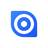is there a way to ''exclude'' the actual field to avoid circular reference in formula field?

I have a field : Group

then I have a formula field and I wish to add a formula to set the right group...

let t := this;
if cnt((select Achats)[text(Livraison) = "Sous-Groupe" and number(Atelier.ID) = number(t.Atelier.ID) and text(group) = "A"]) = 0 then "A"

else

same with ''B"....

So I count the number of ''A'' and if 0 then the field = A; if cnt ''B'' = 0 then B etc...

this way, the field will take the next availlable value. But the problem is that inside the formula, I use the field ''group'' and it lead to a circular reference... how can i exclude the actual field to avoid this circular reference?

thaks!Hi,

That you check the field "group" in other records in your formula should not lead to a circular reference as you compare it with a simple text.

`let myAtel := number(Atelier.ID);`
`if cnt((select Achats)[text(Livraison) = "Sous-Groupe" and number(Atelier.ID) = myAtel and text(group) = "A"]) = 0 then "A"`

`else`

`''B"`

`end`

Best, Jörg